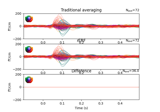# mne.stats.linear_regression_raw#

mne.stats.linear_regression_raw(raw, events, event_id=None, tmin=-0.1, tmax=1, covariates=None, reject=None, flat=None, tstep=1.0, decim=1, picks=None, solver='cholesky')[source]#

Estimate regression-based evoked potentials/fields by linear modeling.

This models the full M/EEG time course, including correction for overlapping potentials and allowing for continuous/scalar predictors. Internally, this constructs a predictor matrix X of size n_samples * (n_conds * window length), solving the linear system `Y = bX` and returning `b` as evoked-like time series split by condition. See 1.

Parameters
rawinstance of `Raw`

A raw object. Note: be very careful about data that is not downsampled, as the resulting matrices can be enormous and easily overload your computer. Typically, 100 Hz sampling rate is appropriate - or using the decim keyword (see below).

events`ndarray` of `int`, shape (n_events, 3)

An array where the first column corresponds to samples in raw and the last to integer codes in event_id.

event_id

As in Epochs; a dictionary where the values may be integers or iterables of integers, corresponding to the 3rd column of events, and the keys are condition names. If None, uses all events in the events array.

tmin

If float, gives the lower limit (in seconds) for the time window for which all event types’ effects are estimated. If a dict, can be used to specify time windows for specific event types: keys correspond to keys in event_id and/or covariates; for missing values, the default (-.1) is used.

tmax

If float, gives the upper limit (in seconds) for the time window for which all event types’ effects are estimated. If a dict, can be used to specify time windows for specific event types: keys correspond to keys in event_id and/or covariates; for missing values, the default (1.) is used.

covariatesdict-like | `None`

If dict-like (e.g., a pandas DataFrame), values have to be array-like and of the same length as the rows in `events`. Keys correspond to additional event types/conditions to be estimated and are matched with the time points given by the first column of `events`. If None, only binary events (from event_id) are used.

reject

For cleaning raw data before the regression is performed: set up rejection parameters based on peak-to-peak amplitude in continuously selected subepochs. If None, no rejection is done. If dict, keys are types (‘grad’ | ‘mag’ | ‘eeg’ | ‘eog’ | ‘ecg’) and values are the maximal peak-to-peak values to select rejected epochs, e.g.:

```reject = dict(grad=4000e-12, # T / m (gradiometers)
mag=4e-11, # T (magnetometers)
eeg=40e-5, # V (EEG channels)
eog=250e-5 # V (EOG channels))
```
flat

For cleaning raw data before the regression is performed: set up rejection parameters based on flatness of the signal. If None, no rejection is done. If a dict, keys are (‘grad’ | ‘mag’ | ‘eeg’ | ‘eog’ | ‘ecg’) and values are minimal peak-to-peak values to select rejected epochs.

tstep`float`

Length of windows for peak-to-peak detection for raw data cleaning.

decim`int`

Decimate by choosing only a subsample of data points. Highly recommended for data recorded at high sampling frequencies, as otherwise huge intermediate matrices have to be created and inverted.

picks

Channels to include. Slices and lists of integers will be interpreted as channel indices. In lists, channel type strings (e.g., ```['meg', 'eeg']```) will pick channels of those types, channel name strings (e.g., `['MEG0111', 'MEG2623']` will pick the given channels. Can also be the string values “all” to pick all channels, or “data” to pick data channels. None (default) will pick good data channels. Note that channels in `info['bads']` will be included if their names or indices are explicitly provided.

solver

Either a function which takes as its inputs the sparse predictor matrix X and the observation matrix Y, and returns the coefficient matrix b; or a string. X is of shape (n_times, n_predictors * time_window_length). y is of shape (n_channels, n_times). If str, must be `'cholesky'`, in which case the solver used is `linalg.solve(dot(X.T, X), dot(X.T, y))`.

Returns
evokeds`dict`

A dict where the keys correspond to conditions and the values are Evoked objects with the ER[F/P]s. These can be used exactly like any other Evoked object, including e.g. plotting or statistics.

References

1

Nathaniel J. Smith and Marta Kutas. Regression-based estimation of ERP waveforms: II. Nonlinear effects, overlap correction, and practical considerations: rERPS II. Psychophysiology, 52(2):169–181, 2015. doi:10.1111/psyp.12320.

## Examples using `mne.stats.linear_regression_raw`#Regression on continuous data (rER[P/F])

Regression on continuous data (rER[P/F])